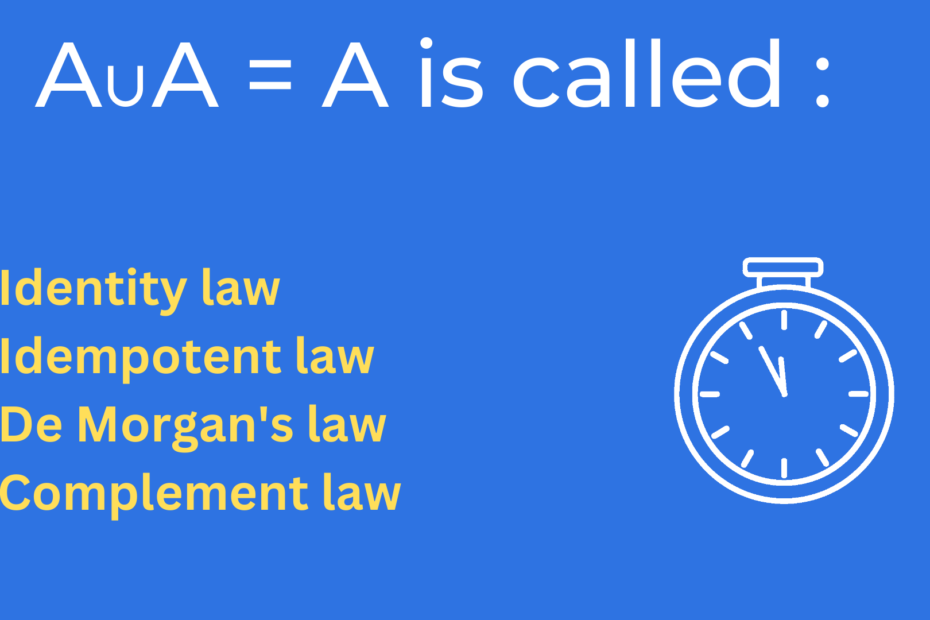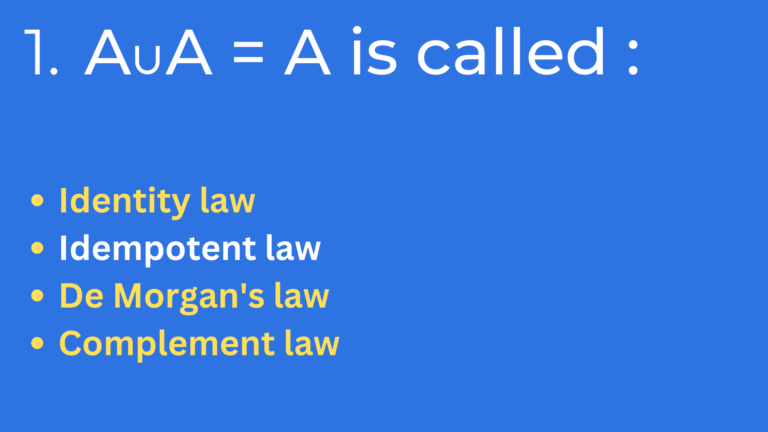# AUA = A is called

• CS PYQ, PYQ## AUA = A is called :

• Identity law
• Idempotent law
• De Morgan’s law
• Complement law

## Identity law

• The identity law is a basic principle in mathematics that states that for any value, “a”, it is always true that “a” equals “a”.
• In other words, the identity law states that any value is equal to itself.
• This law is often written as “a = a” or “a ≡ a”.
• The identity law is one of the fundamental laws of algebra and is used as a basis for many other mathematical principles and equations.
• It is also known as the reflexive property, as it states that any value has a reflexive relationship with itself.

## Idempotent law

• The idempotent law states that when a function or operation is applied to a value multiple times, the result will always be the same as if it was applied only once. This means that if a function or operation f is applied to a value x, and we apply it again to the result of the first application, we will get the same result as if we only applied it once.

• Mathematically, this can be represented as:

f(f(x)) = f(x) or AUA = A

• This law is important in programming, as it ensures that certain operations can be performed repeatedly without changing the outcome. It is also used in mathematics and computer science to prove certain properties of functions and operations.

## De Morgan's law

• De Morgan’s law is a logical principle that states that the negation of a conjunction is the disjunction of the negations, and the negation of a disjunction is the conjunction of the negations.

• This can be expressed mathematically as:

Not (A and B) = (not A) or (not B)

Not (A or B) = (not A) and (not B)

• This law is named after Augustus De Morgan, a 19th century British mathematician and logician. It is often used in Boolean algebra and logic to simplify complex logical statements and to prove logical equivalences.

## Complement law

• The complement law is a mathematical principle that states that the probability of an event occurring is equal to 1 minus the probability of it not occurring.
• In other words, if the probability of an event occurring is p, then the probability of it not occurring is 1-p.
• The union of a set A and its complement A’ gives the universal set U of which, A and A’ are a subset. A ∪ A’ = U
• This law is often used in probability and statistics to calculate the probability of an event occurring given certain conditions or variables.
• It is also commonly used in decision-making and risk assessment to evaluate the likelihood of an event occurring.

Hence, we can say that  AUA = A is called Idempotent law